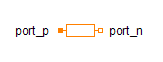Linear Chirp - MapleSim Help

Linear Chirp

Generate a chirp whose frequency which varies linearly with timeDescription The Linear Chirp component generates a constant amplitude chirp whose instantaneous frequency, $f$, varies linearly with time. The chirp begins at time ${T}_{0}$ and ends after duration ${T}_{d}$. The instantaneous frequency varies from ${f}_{1}$ to ${f}_{2}$.Equations $f={f}_{1}+k\left(t-{T}_{0}\right)$ ${\mathrm{int}}_{f}={f}_{1}\left(t-{T}_{0}\right)+\frac{1}{2}k{\left(t-{T}_{0}\right)}^{2}$ $y={y}_{0}+\left\{\begin{array}{cc}A\mathrm{sin}\left(2\pi {\mathrm{int}}_{f}\right)& {T}_{0}\le t\le {T}_{0}+{T}_{d}\\ 0& \mathrm{otherwise}\end{array}$Connections

 Name Description Modelica ID $y$ Real output signal y $f$ Instantaneous frequency fParameters

 Name Default Units Description Modelica ID ${y}_{0}$ $0$ Offset of output signal offset $A$ $1$ Amplitude of sine wave amplitude ${f}_{1}$ $1$ $\mathrm{Hz}$ Initial frequency freqHz1 ${f}_{2}$ $10$ $\mathrm{Hz}$ Final frequency freqHz2 ${T}_{0}$ $0$ $s$ Start of chirp startTime ${T}_{d}$ $2$ $s$ Duration of chirp durationModelica Standard Library The component described in this topic is from the Modelica Standard Library. To view the original documentation, which includes author and copyright information, click here.